/

### Finding The Most Important Principal Component

Suppose we have a set ofseries of returns (or losses, …). A principal component is a set of exposures (and a principal component series is a series of returns) corresponding to an eigenvector of the relevant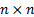covariance matrix,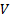. Eigenvectors satisfy the vector equation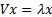for some scalar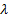.

Typically principal components are identified in practice using suitable software packages designed to identify eigenvectors and eigenvalues, applied to the relevant covariance matrix,, e.g. using using Nematrian web services functions that target principal components, i.e. MnPrincipalComponents, MnPrincipalComponentsSizes and MnPrincipalComponentsWeights.

However,  for the first, i.e. most important, principal component there is a conceptually simpler approach as follows.

We note that any vector can be written as a combination of the eigenvectors of a matrix, and that these eigenvectors can be chosen to be orthonormal (if suitably chosen if some eigenvalues take the same value) so we can write any vector,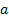, of active positions as the sum of positions,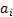, in the relevant eigenvectors,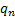, i.e. as: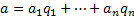Then,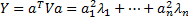. If we order the eigenvectors (principal components) so that the most important ones are first, i.e.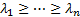then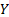is maximised, subject to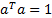, if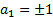and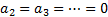. Thus, we can identify the most important principal component by reference to the set of positions of unit magnitude that exhibit the largest risk (here equated with ex-ante tracking error/variance/VaR).